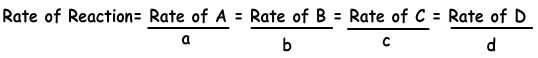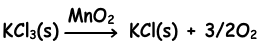Rates of Reactions (Chemical Kinetics)

Rates of Reactions (Chemical Kinetics)

Physical, chemical and nuclear reactions take place in different speeds. Chemical rate is the amount of change in the matter in unit time.

Reaction Rate=(Change in amount of matter)/time

Δ[A(g)] is the representation of change in molarity of A gas. Reaction rate can be written for all matters in the reaction.

Example:

N2(g) + 3H2(g) → 2NH3

Reaction rates for N2, H2 and NH3 is given below;

Reaction Rate of N2= - Δ[N2(g)]/Δt

Reaction Rate of H2= - Δ[H2(g)]/Δt

Reaction Rate of NH3=  Δ[NH3(g)]/Δt

"-" sign in front of N2 and H2 gases show that these matters are used in reaction and sign of NH3 is positive since it is produced in reaction. A chemical reaction, of course has one reaction rate. Following equation shows reaction rate in terms of products and reactants.

aA + bB → cC + dDIf we write rate of reaction for NH3;

Rate of Reaction= Rate of N2/1 = Rate of H2/3 = Rate of NH3/2

As we give before we can define rate of reaction as;

Reaction Rate=(Change in amount of matter)/time

Example: Electrolysis of 18 g water takes 10 minutes. Find;

I. Rate of formation of H2

II. Rate of formation of O2

III. Rate of consumption of H2O

Solution:

Rate of formation of H2 = +2/10=0,2 g/minute

Rate of formation of O2 = +16/10=1,6 g/minute

Rate of consumption of H2O = -18/10= - 1,8 g/minute

Activation Energy

It is the energy required to start chemical reaction. Reactions having higher activation energy has lower reaction rate. Activation energy can only be changed by catalysts. They decreases activation energy of reaction and increase rate of reaction.MnO2 is the catalyst of this reaction.

Factors Affecting Reaction Rate

• Types of matters in reaction
• Concentrations and physical states of matters in reaction
• Temperature
• Pressure and volume
• Catalysts
• Surface of contact

Example: Which ones of the following statements should be done to increase rate of formation of CO2 gas in following reaction.

C(s) + O2(g) → CO2(g)

I. Making C(s) powder

III. Adding CO2 gas into container

Solution:

To increase rate of reaction, making matters gas, increasing temperature, decreasing volume, using catalysts, increasing surface of contact should be done. On the other hand, adding reactants do not affect reaction rate.

Rates of Reaction Exams and Problem Solutions

Related